8.5. Calculation of the Spectrum of the Lithium Atom

The lithium atom has three electrons. Lithium containing one electron is considered to be a hydrogen-like atom. We have already shown how the spectra of the hydrogen-like atoms are calculated, including the hydrogen-like lithium atom (Table 9). Let us calculate the spectrum of the second electron of this atom.

Ionization energy of the second electron of the lithium atom is equal to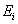=75.638 eV. According to Pauli-Fermi principle, the second electron cannot occupy the first energy level , because it has already been occupied by the electron of the hydrogen-like atom (the third electron). That’s why it is necessary to find binding energy of the second electron of the lithium atom corresponding to the second energy level. For this purpose, let us write from the reference book a row of experimental values of excitation energies corresponding to stationary energy levels of this electron : 62.41; 69.65; 72.26; 73.48; …. eV.

As the second electron of the lithium atom cannot occupy the first energy level, the first excitation energy of 62.41 eV in a variety of excitation energies corresponding to the stationary energy levels should belong to the second energy level of this electron. Let us find a difference between ionization energy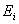=75.638 eV of this electron and excitation energy corresponding to the second energy level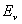=62.41 eV.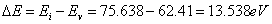(221)

Now let us multiply the difference DE by square of the main quantum number corresponding to the second energy level: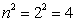. The result being obtained will correspond to binding energy of the second electron of the lithium atom with the atomic nucleus at the time of its stay on the first energy level. In accordance with Pauli-Fermi principle, this electron cannot occupy the first energy level, that’s why the energy being obtained, which corresponds to this level, will be fictitious. Here is its value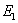=13.538×4=54.152 eV.

Thus, ionization energy of=75.638 eV of the second electron of the lithium atom is not equal to energy of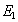=54.152 eV of its binding with the atomic nucleus corresponding to the first energy level. If we insert these data into the formulas (206) and (207), we’ll get (Table 14).

Table 14.  Spectrum of the second electron of the lithium atom

 Valumes n 2 3 4 5 6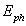(exper) eV 62.41 69.65 72.26 73.48 -(theor.) eV 62.41 69.62 72.25 73.47 74.13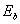(theor.) eV 13.54 6.02 3.38 2.17 1.50

Let us calculate the spectrum of the first electron of the lithium atom. Its ionization energy is=5.392 eV, and a row of excitation energies corresponding to the stationary energy levels is as follows : 3.83; 4.52; 4.84; 5.01; 5.11; 5.18; 5.22; 5.25; 5.28; 5.30; 5.31 eV.

The difference between ionization energy of this electron and excitation energy corresponding to the third stationary energy level will be as follows: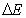=5.39-3.83=1.5 eV. Then we’ll find binding energy of this electron with the atomic nucleus corresponding to the first fictitious energy level.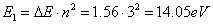.                                       (222)

Thus, ionization energy of the first electron of the lithium atom is=5.392 eV, and fictitious binding energy with the nucleus corresponding to the first energy level is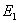=14.05 eV. If we insert these data into the mathematical model of formation of the spectra of the atoms and the ions (207) and into the formula (206) of the calculation of  binding energies of  this electron corresponding to the stationary energy levels, we’ll get a spectrum of this electron (Table 15).

Table 15.  Spectrum of the first electron of the lithium atom

 Volumes N 2 3 4 5 6(exper) eV - 3.83 4.52 4.84 5.01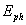(theor.) eV 62.41 3.83 4.51 4.83 5(theor.) eV 3.51 1.56 0.88 0.56 0.39

8.6. Calculation of the Spectrum of the Beryllium Atom

The beryllium atom has four electrons. The fourth electron has the largest ionization energy, the first electron has the smallest ionization energy. We’ll not give the calculation of the spectrum of the fourth electron of this atom, because its results are given in Table 6 as a spectrum of the hydrogen-like atom. We’ll not repeat completely the details of the calculation method of the spectra of the third, the second and the first electrons of this atom, we’ll give only the key points of this method.

Ionization energy of the third electron of the beryllium atom is equal to=153.893 eV. Excitation energies of this electron corresponding to the stationary energy levels make the following row : 123.67; 140.39; 146.28; 149.01; 150.50; 151.40 eV. The difference between ionization energy and the value of the first energy in this row will be equal to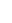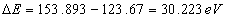.                                                            (223)

Binding energy of the third electron with the atomic nucleus corresponding to the first energy level is determined in such a way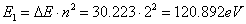(224)

If we insert the values=153.893 eV and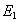=120.892 eV into the formulas (206) and (207), well find (Table 16).

Table 16. Spectrum of the third electron of the beryllium atom

 Valumes n 2 3 4 5 6(exper) eV 123.7 140.4 146.3 149 150.5(theor.) eV 123.7 140.5 146.3 149 150.5(theor.) eV 30.22 13.43 7.56 4.84 3.36

The second electron of the beryllium atom has ionization energy=18.211 eV and the following row of excitation energies corresponding to the stationary energy levels : 3.96; 11.96; 14.7; 15.99; 16.67; 17.08 eV.

Let us pay attention to the fact that the energy value of 3.96 eV exceeds the limits of the excitation energy formation regularity supposed by us. In the reference book  this spectral line is given as a bright line, that’s why we have every reason to take it into consideration. We have one opportunity: we can suppose that the second electron of the beryllium atom can have two positions in the atom, and it is connected with the structure of its nucleus. Later on, we’ll analyze the structures of the atomic nuclei and try to find the answer to this ambiguity. Now we have only one possibility: we should suppose that excitation energy 3.96 eV and the remaining energies 11.96; 14.7; 15.99; 16.67; 17.08 eV correspond to various positions of the second electron in the atom, that’s; why we try to get theoretically only the row 11.96; 14.7; 15.99; 16.67; 17.08 eV. For this purpose, we’ll find the difference between ionization energy=18.211 eV and the energy 11.96 eV.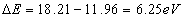.                                       (225)

The binding energy of the second electron of the beryllium atom corresponding to the first fictitious energy level will be:=6.25×9=56.259 eV. If we insert this value  and ionization energy=18.211 eV into the formulas (206) and (207), we’ll find (Table 17).

Table 17.  Spectrum of the second electron of the beryllium atom

 Values n 2 3 4 5 6(exper) eV - 11.96 14.72 15.99 16.67(theor.) eV 4.15 11.96 14.7 15.96 16.65(theor.) eV 14.81 6.25 3.52 2.25 1.56

The theory predicts (Table 17) the existence of excitation energy of 4.15 eV corresponding to the second energy level, but it seems that its is fictitious value of energy.

The first electron of the beryllium atom has ionization energy of=9.322 eV and the following row of excitation energies : 2.73; 5.28; 7.46; 8.31; 8.69 eV. We’d like to note that there is no energy of 2.73 eV in the reference book , and in the reference book  it is given without specification of its brightness. It allows us not to take it into consideration. Thus, difference of energies will be equal to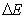=9.322-5.28=4.04 eV, and energy corresponding to the first fictitious energy level will be as follows: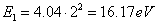. If we insert=9.322 eV and=16.17 eV in the formulas (206) and (207), we’ll find (Table 18).

Table 18. Spectrum of the first electron of the beryllium atom

 Values n 2 3 4 5 6 7 8(exper) eV 5.28 7.46 8.31 8.69 8.86 8.98 9.07(theor.) eV 5.28 7.53 8.31 8.67 8.87 8.99 9.07(theor.) eV 4.04 1.8 1.01 0.65 0.45 0.33 0.25

Note: experimental values of excitation energies corresponding to the 6th, 7th and 8th energy levels are taken from the reference book .

The mathematical models (206) and (207) gave satisfactory results. But they were the spectra of the atoms and the ions of the first four elements of the periodic law of the chemical elements. They are the simples atoms.

If the electrons actually presses on the atomic nuclei, they begin to interact when their quantity is increased in the atom, and the mathematical models fail to take it into consideration (206) and (207). There is every reason to suppose that in the atoms with a large number of electrons in these models the correction factors or trigonometric functions will appear, which can characterize the arrangement of a cell of the electron in the atom. The cell is considered to be a cavity of a conic form, in which basis the electron is situated, and the vertex is directed to the atomic nucleus.

A question arises: what aim can be when the spectra of the atoms and the ions are calculated? The fist aim is to get the information for the determination of the structure of the atom and its nucleus. The second aim is to calculate bindings of valency electrons with the atomic nuclei in order to use them for the analysis of energy balance in various chemical reactions. The first aim is a remote one; nevertheless, we make the first steps to this aim. The second aim is nearer to practice, that’s why it deserves a priority attention. Taking these facts into consideration we’ll try to calculate the spectra of valency electrons, which have the smallest ionization energies.

Later on during the analysis of the structure of the atomic nuclei and the atoms of chemical elements, we’ll see that all electrons are in the atom, their binding energies with the protons of the nuclei are nearly the same.

We have already agreed that we call an electron with the smallest ionization potential the first electron. This electron is called valency electron. Later on we’ll see that the atomic nuclei have equal potential possibilities to be valency electrons. That’s why the enumeration of the electrons in the atom is a conditional thing. Let us try to calculate the spectrum of the electron of boron atom, which has the smallest ionization potential. Let us call this electron the first electron.

8.7. Calculation of the Spectrum of the First Boron Atom

The boron atom has five electrons. Let us call the electron, which has the least ionization energy=8.298 eV, the first electron. It has the following row of excitation energies: 4.96; 5.93; 6.79; 6.82; 7.44; 7.46; 7.75; 7.88; 7.92; 7.95; 8.02; 8.03; 8.08; 8.09; 8.13; 8.16; 8.18; 8.20; 8.22; 8.23; 8.24; 8.25; 8.26; 8.27 eV. The row is rather long. Let us pay attention to the underlined close values of energies. It seems they are doublets and triplets, i.e. the slip atoms. That’s why the calculation should give one of the underlined values or their average values. Let us see if it is so or not. The difference of energies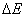=8.298-4.96=3.34 eV. Binding energy of this electron with the atomic nucleus corresponding to the first fictitious energy level is determined according to the formula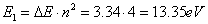=DE×22=3.34× 4=13.35 eV. If we insert=8.298 eV and=13.35 eV in the formulas (206) and (207), we’ll find (Table 19).

Table 19. Spectrum of the first electron of the boron atom

 Valumes n 2 3 4 5 6 7(exper) eV 4.96 6.82 7.46 7.75 7.92 8.02(theor.) eV 4.96 6.81 7.46 7.76 7.93 8.02 Valumes n 8 9 10 11 12 13(exper) eV 8.09 8.13 8.16 8.18 8.20 8.22(theor.) eV 8.09 8.13 8.16 8.18 8.20 8.22 Valumes n 14 15 16 17 18 19(exper) eV 8.23 8.24 8.25 8.25 8.26 ...(theor.) eV 8.23 8.24 8.25 8.25 8.26 ...

If we analyse the given experimental row of excitation energies and the results of its calculation given in Table 19, we can see a good convergence of theoretical and experimental data.

Later on we’ll not try to calculate the spectra of all electrons and all atoms, we’ll give only the calculations of the spectra of the atoms and those valency electrons, which we have used during the analysis of the results of our investigations.

The Foundations of Physchemistry of Microworld

Ó2003 Kanarev Ph. M.

Internet Version - http://book.physchemistry.innoplaza.net

<< Back to Physchemistry Book Index